Next: Related Work Up: Index Previous: Abstract

# Introduction

Usually the goal of volume visualization is to get meaningful, two-dimensional pictures from three-dimensional data sets (for instance from CT or MRI imaging). A useful concept for that purpose is the gradient, which can be interpreted as a normal to a surface of equal values (an iso-surface) passing through the point of interest. Consequently, the gradient can be used to shade surfaces, since most lighting models (for instance Gouraud shading  or Phong shading ) make use of it or it can be used for classification in ray casting techniques . Möller et al. even concede gradient reconstruction having a greater impact on image quality than function reconstruction itself .

From signal processing theory we know that the ideal reconstruction filter is the sinc function  given by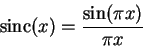(1)

Provided that the function was sampled properly (according to Shannons sampling theorem, which states that the sampling frequency must be at least twice the highest frequency present in the function ) it can be reconstructed exactly by convolving (denoted by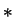) the samples with the sinc function:
 fr(x) =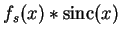(2) =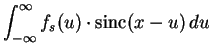=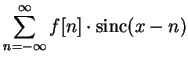where fr(x) denotes the reconstructed function, fs(x) the sampled function and f[n] the samples.

Sampling and reconstruction are often investigated in frequency domain. A function can be transformed by means of the Fourier transformation from the spatial domain to the frequency domain, where additional information about its behavior can be obtained (reconstruction filters, for instance, can be compared to the ideal one, i.e., the sinc filter). An excellent introduction to this topic is given by Blinn [2,3]. In order to reconstruct the derivative of the function it is possible to reconstruct the function and derive it discretely. However, we chose another approach which is to derive the ideal reconstruction filter and use it as a derivative reconstruction filter directly.

Deriving the sinc function (Eq. 1) yields the cosc function  (note that the definition is not analogous):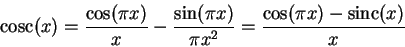(3)

The frequency response of the cosc function is depicted together with the frequency response of the sinc function in Fig. 1 on the right, whereas the functions itself are depicted on the left. Since both sinc and cosc filter are of infinite extend we have to use in practice other, finite, filters which can, obviously, never reach the optimal frequency response. However, we can compare them to the optimal one and, consequently, gain additional information about their quality.

In this paper we will compare various filters obtained by bounding the ideal derivative reconstruction filter given by Eq. 3 to some finite extend. The simplest way to do this is to truncate the cosc function, which is, anyway, not the best way because of discontinuities at the edges. So special functions (adversely called windows) were designed to get a smoother transition to zero. Their definitions and a discussion of their frequency responses is given in Section 3 after a brief overview of some work already done in this area in Section 2. In Section 4 the test scenario used to compare the windowed cosc filters is described and the result are given and discussed in Section 5.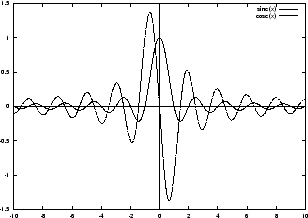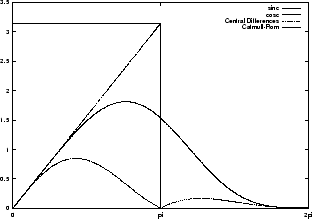Next: Related Work Up: Index Previous: Abstract

1999-04-08